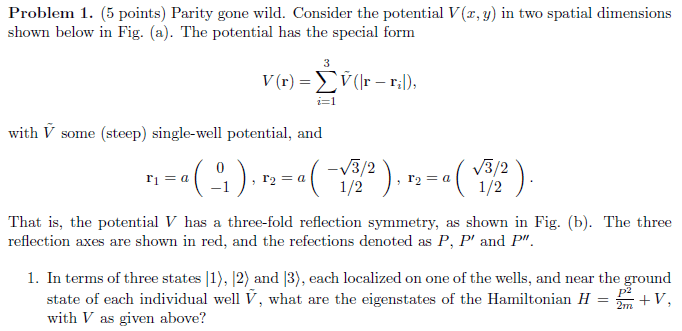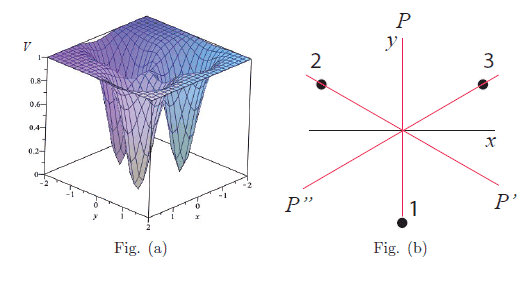# A potential well with 3-fold reflection symmetry

lazayama
Homework Statement:
Photos are attached below, with figures
Relevant Equations:
Bloch's theorem: $$\psi(r)=e^{ikr}u(r)$$
Simultaneous eigenstates of Hamiltonian and Translational operator: $$|\theta>=\Sigma_{n=0} ^{N-1}|n>*e^{in\theta}$$
When we are talking about Bloch's theorem and also the tight-binding approximation, we can use them to help finding eigenstates of a system. However, I am so confused how to apply it in this case (below is my homework) and don't even know how to start it...

All I understand about the Bloch's theorem is that we can find a wavefunction that is a product of plane wave and a periodic function.

This will be great if someone can discuss it with me and direct my thinking...This may be a silly question but I am really lost here... I am not asking for direct answer but I really need a hand on this, so a solid discussion would be really helpful.